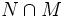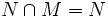# Normality-large and minimal normal implies monolith

## Statement

A Minimal normal subgroup (?) of a group that is normality-large, must be a Monolith (?): it is contained in every nontrivial normal subgroup.

## Facts used

1. Normality is strongly intersection-closed

## Proof

Given: A group$G$, a minimal normal subgroup$N$ such that if$N \cap M$ is trivial for any normal subgroup$M$, then$M$ is trivial.

To prove: For any normal subgroup$M$, either$M$ is trivial or$N \le M$.

Proof: Since both$N$ and$M$ are normal, fact (1) tells us that$N \cap M$ is normal. So$N \cap M$ is a normal subgroup of$G$ contained in a minimal normal subgroup$N$. So there are two cases:

•$N \cap M = N$: In this case,$N \le M$.
•$N \cap M$ is trivial: In this case, the normality-largeness of$N$ tells us that$M$ is trivial.

This completes the proof.